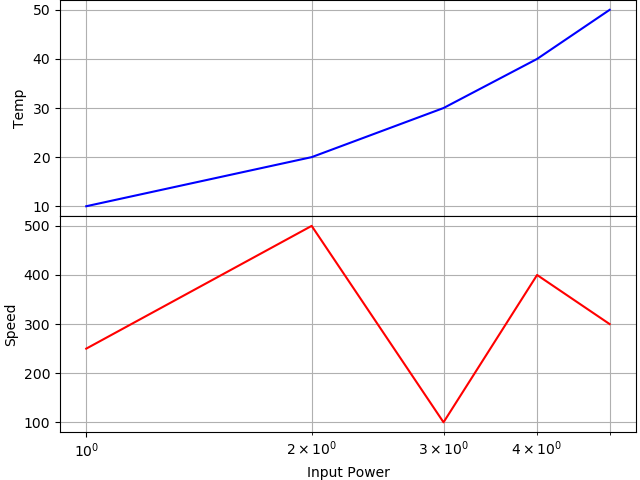# Unable to hide xticklabels when two subplots share x axis

I am trying to create a graph where the two subplots share the same x-axis. Below is my example code. I am having the following questions and issues:

1. In my example code, hiding the xticklabels for ax1 doesn’t do anything. Only the first tick label disappears but the remaining still stay.2. I would like to close the gap between the two subplots. I do this with hspace=0. However, this setting is not respected when I use tight_layout().

3. What is the difference between method 1 and method 2 to create subplots with shared x-axis. Both seem to result in different behavior. For example, if I use method 1, there is no xticklabels on ax1, but the xlabel appears visible. If I use method 2, xticklabels appear, but xlabel disappears.

4. After I set hspace=0, how do I correct the almost overlap yticklabels? https://imgur.com/5w4xt9J.png

``````import matplotlib.pyplot as plt
import numpy as np
from matplotlib import gridspec
%matplotlib notebook

# Method 1
# fig, (ax1, ax2) = plt.subplots(2,1, sharex=True,)

# Method 2
fig = plt.figure()
gs = gridspec.GridSpec(2, 1,)
ax1 = plt.subplot(gs)
ax2 = plt.subplot(gs, sharex = ax1)

# First subplot
ax1.plot([1,2,3,4,5], [10,20,30,40,50], color='b')
ax1.set_yscale('linear')
ax1.set_ylabel( 'Temp' )
ax1.set_xscale('log')
ax1.set_xlabel( 'Input Power' )

# Second subplot
ax2.plot([1,2,3,4,5], [250,500,100,400,300], color='r')
ax2.set_yscale('linear')
ax2.set_ylabel( 'Speed' )
ax2.set_xscale('log')
ax2.set_xlabel( 'Input Power' )

plt.setp(ax1.get_xticklabels(), visible=False)
# plt.setp(ax2.get_xticklabels(), visible=False)
# plt.tight_layout()

``````

Will respond to your questions later (or maybe someone else can try to help)…

Here is a quick solution to what you are trying to do…

``````%matplotlib notebook
import matplotlib.pyplot as plt

fig, (ax1, ax2) = plt.subplots(2, 1, sharex=True, constrained_layout=True)
ax1.plot([1, 2, 3, 4, 5], [10, 20, 30, 40, 50], color='b')
ax2.plot([1, 2, 3, 4, 5], [250, 500, 100, 400, 300], color='r')
ax1.set_ylabel('Temp')
ax1.set_xscale('log')  # don't need to set xscale for ax2 becuase sharex=True
ax1.tick_params(axis='x', which='both',
bottom=False) # turn off major & minor ticks on the bottom
ax2.set_ylabel('Speed')
ax2.set_xlabel('Input Power')
ax1.grid(which='both') # turning on both major and minor grid
ax2.grid(which='both')
``````1 Like

The reason that `plt.setp(ax1.get_xticklabels(), visible=False)` behaves is because we do some complicated caching / just in time generation of the `Text` objects used for the tick labels. When you are calling it, all of the text objects do not exist yet (but the first one does!).

The way that @pharshalp suggests is the idiomatic way to turn off the ticks and tick labels.

 mostly performance and historical

1 Like

Problem: I need to use latex to have a superscript in the xlabel. When I use latex in the xlabel, part of the xlabel gets cut off. I am unable to use `fig.tight_layout()`, to fix the issue.

``````%matplotlib widget
import matplotlib.pyplot as plt
import matplotlib as mpl
import numpy as np
import seaborn as sns

# I am paranoid so first set the style to defaults
mpl.style.use('default') # https://matplotlib.org/3.1.1/api/_as_gen/matplotlib.pyplot.rc.html

# Now set style using seaborn
sns.set()
sns.set_context('notebook')
sns.set_style('ticks')

fig, (ax1, ax2) = plt.subplots(2, 1, sharex=True, constrained_layout=True)

# Get ax2 ticks to show on top so it looks like its part of ax1 ticks
ax2.tick_params(
axis='x',           # changes apply to the x-axis
which='both',       # both major and minor ticks are affected
direction='inout',
# bottom=True,
top=True,
labelbottom=True,    # labels along the bottom edge are on
length=5,
)

ax1.plot([1, 2, 3, 4, 5], [10, 20, 30, 40, 50], color='r')
ax2.plot([1, 2, 3, 4, 5], [250, 500, 100, 400, 300], color='b')
ax1.set_xscale('log')  # don't need to set xscale for ax2 becuase sharex=True
ax1.set_ylabel('Temp')
# ax1.set_xlabel('Intensity (mW/cm\$^2\$)')
ax2.set_ylabel('Speed')
ax2.set_xlabel('Intensity (mW/cm\$^2\$)')  # When using \$^2\$, the xlabel gets cut off
# ax2.set_xlabel('Intensity (mW/cm2)')     # When NOT using \$^2\$, the xlabel does NOT get cut off

# fig.tight_layout()
``````

The cutoff of the label is due to https://github.com/matplotlib/matplotlib/issues/15313 which is still not solved.
The only solution is to add some space to the bottom of the figure. For that you need to understand that constrained_layout and tight_layout are mutually exclusive.
Unfortunately, constrained_layout cannot help here, because its padding and spacing parameters are coupled, so you cannot have 0 spacing between the plots, but non-zero padding.
This leaves us with tight_layout.
A solution would then look like

``````import matplotlib.pyplot as plt
import seaborn as sns

plt.style.use('default')
sns.set()
sns.set_context('notebook')
sns.set_style('ticks')

fig, (ax1, ax2) = plt.subplots(2, 1, sharex=True, constrained_layout=False)

ax2.tick_params( axis='x',  which='both', direction='inout', top=True,
labelbottom=True, length=5, )

ax1.plot([1, 2, 3, 4, 5], [10, 20, 30, 40, 50], color='r')
ax2.plot([1, 2, 3, 4, 5], [250, 500, 100, 400, 300], color='b')
ax1.set_xscale('log')
ax1.set_ylabel('Temp')
ax2.set_ylabel('Speed')
ax2.set_xlabel('Intensity (mW/cm\$^2\$)')

fig.tight_layout()